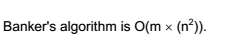# Banker’s Algorithm (Dijkstra and Habermann) A+ A  A-

Banker’s Algorithm (Dijkstra and Habermann):It is a deadlock avoidance algorithm.

The following data structures are used in the algorithm:
m = number of resources
n = number of processes

Available [m] : One dimensional array of size m. It indicates the number of available resources of each type. For example, if Available [i] is k, there are k instances of resource ri.

Max [n,m] : Two dimensional array of size n*m. It defines the maximum demand of each process from each resource type. For example, if Max [i,j] is k, process pi may request at most k instances of resource type rj.

Allocation [n,m] : Two dimensional array of size n*m. It defines the number of resources of each type currently allocated to each process.

Need [n,m] : Two dimensional array of size n*m. It indicates the remaining need of each process, of each resource type. If Need [i,j] is k, process pi may need k more instances of resource type rj. Note that Need [i,j] = Max [i,j] – Allocation [i,j].

Request [n,m] : Two dimensional array of size n*m. It indicates the pending requests of each process, of each resource type.

Now, take each row vector in Allocation and Need as Allocation(i) and Need(i). (Allocation(i) specifies the resources currently allocated to process pi. )

Define the ≤ relation between two vectors X and Y , of equal size = n as :
X ≤ Y ⇔ X [ i ] ≤ Y [ i ] , i = 1,2, …, n
X !≤ Y ⇔ X [ i ] > Y [ i ] for some i

The algorithm is as follows:

1. Process pi makes requests for resources. Let Request(i) be the corresponding request vector. So, if pi wants k instances of resource type rj, then Request(i)[j] = k.
2. If Request(i) !≤ Need(i), there is an error.
3. Otherwise, if Request(i) !≤ Available, then pi must wait.
4. Otherwise, Modify the data structures as follows :
Available = Available – Request(i)
Allocation(i) = Allocation(i) + Request(i)
Need(i) = Need(i) – Request(i)
5. Check whether the resulting state is safe. (Use the safety algorithm presented below.)
6. If the state is safe, do the allocation. Otherwise, pi must wait for Request(i).

Safety Algorithm to perform Step 5:

Let Work and Finish be vectors of length m and n, respectively.
1. Initialize Work = Available, Finish [j] = false, for all j.
2. Find an i such that Finish [ i ] = false and Need(i) ≤ Work If no such i is found, go to step 4.
3. If an i is found, then for that i, do :
Work = Work + Allocation(i)
Finish [i] = true
Go to step 2.
4. If Finish [j] = true for all j, then the system is in a safe state.